This function performs a $$p$$-curve analysis using a meta object or calculated effect size data.

pcurve(x, effect.estimation = FALSE, N, dmin = 0, dmax = 1)

## Arguments

x Either an object of class meta, generated by the metagen, metacont, metacor, metainc, or metabin function, or a dataframe containing the calculated effect size (named TE, log-transformed if based on a ratio), standard error (named seTE) and study label (named studlab) for each study. Logical. Should the true effect size underlying the p-curve be estimated? If set to TRUE, a vector containing the total sample size for each study must be provided for N. FALSE by default. A numeric vector of same length as the number of effect sizes included in x specifying the total sample size $$N$$ corresponding to each effect. Only needed if effect.estimation = TRUE. If effect.estimation = TRUE: lower limit for the effect size ($$d$$) space in which the true effect size should be searched. Must be greater or equal to 0. Default is 0. If effect.estimation = TRUE: upper limit for the effect size ($$d$$) space in which the true effect size should be searched. Must be greater than 0. Default is 1.

## Value

Returns a plot and main results of the pcurve analysis:

• P-curve plot: A plot displaying the observed $$p$$-curve and significance results for the right-skewness and flatness test.

• Number of studies: The number of studies provided for the analysis, the number of significant $$p$$-values included in the analysis, and the number of studies with $$p<0.025$$ used for the half-curve tests.

• Test results: The results for the right-skewness and flatness test, including the $$p_{binomial}$$ value, as well as the $$z$$ and $$p$$ value for the full and half-curve test.

• Power Estimate: The power estimate and 95% confidence interval.

• Evidential value: Two lines displaying if evidential value is present and/or absent/inadequate based on the results (using the guidelines by Simonsohn et al., 2015, see Details).

• True effect estimate: If effect.estimation is set to TRUE, the estimated true effect $$\hat{d}$$ is returned additionally.

If results are saved to a variable, a list of class pcurve containing the following objects is returned:

• pcurveResults: A data frame containing the results for the right-skewness and flatness test, including the $$p_{binomial}$$ value, as well as the $$z$$ and $$p$$ value for the full and half-curve test.

• Power: The power estimate and 95% confidence interval.

• PlotData: A data frame with the data used in the $$p$$-curve plot.

• Input: A data frame containing the provided effect sizes, calculated $$p$$-values and individual results for each included (significant) effect.

• EvidencePresent, EvidenceAbsent, kInput, kAnalyzed, kp0.25: Further results of the $$p$$-curve analysis, including the presence/absence of evidence interpretation, and number of provided/significant/$$p<0.025$$ studies.

• I2: $$I^2$$-Heterogeneity of the studies provided as input (only when x is of class meta).

• class.meta.object: class of the original object provided in x.

## Details

P-curve Analysis

$$P$$-curve analysis (Simonsohn, Simmons & Nelson, 2014, 2015) has been proposed as a method to detect $$p$$-hacking and publication bias in meta-analyses.

$$P$$-Curve assumes that publication bias is not only generated because researchers do not publish non-significant results, but also because analysts “play” around with their data ("$$p$$-hacking"; e.g., selectively removing outliers, choosing different outcomes, controlling for different variables) until a non-significant finding becomes significant (i.e., $$p<0.05$$).

The method assumes that for a specific research question, $$p$$-values smaller 0.05 of included studies should follow a right-skewed distribution if a true effect exists, even when the power in single studies was (relatively) low. Conversely, a left-skewed $$p$$-value distribution indicates the presence of $$p$$-hacking and absence of a true underlying effect. To control for "ambitious" $$p$$-hacking, $$P$$-curve also incorporates a "half-curve" test (Simonsohn, Simmons & Nelson, 2014, 2015).

Simonsohn et al. (2014) stress that $$p$$-curve analysis should only be used for test statistics which were actually of interest in the context of the included study, and that a detailed table documenting the reported results used in for the $$p$$-curve analysis should be created before communicating results (link).

Implementation in the function

To generate the $$p$$-curve and conduct the analysis, this function reuses parts of the R code underlying the P-curve App 4.052 (Simonsohn, 2017). The effect sizes included in the meta object or data.frame provided for x are transformed into $$z$$-values internally, which are then used to calculate p-values and conduct the Stouffer and Binomial test used for the $$p$$-curve analysis. Interpretations of the function concerning the presence or absence/inadequateness of evidential value are made according to the guidelines described by Simonsohn, Simmons and Nelson (2015):

• Evidential value present: The right-skewness test is significant for the half curve with $$p<0.05$$ or the $$p$$-value of the right-skewness test is $$<0.1$$ for both the half and full curve.

• Evidential value absent or inadequate: The flatness test is $$p<0.05$$ for the full curve or the flatness test for the half curve and the binomial test are $$p<0.1$$.

For effect size estimation, the pcurve function implements parts of the loss function presented in Simonsohn, Simmons and Nelson (2014b). The function generates a loss function for candidate effect sizes $$\hat{d}$$, using $$D$$-values in a Kolmogorov-Smirnov test as the metric of fit, and the value of $$\hat{d}$$ which minimizes $$D$$ as the estimated true effect.

It is of note that a lack of robustness of $$p$$-curve analysis results has been noted for meta-analyses with substantial heterogeneity (van Aert, Wicherts, & van Assen, 2016). Following van Aert et al., adjusted effect size estimates should only be reported and interpreted for analyses with $$I^2$$ values below 50 percent. A warning message is therefore printed by the pcurve function when x is of class meta and the between-study heterogeneity of the meta-analysis is substantial (i.e., $$I^2$$ greater than 50 percent).

Harrer, M., Cuijpers, P., Furukawa, T.A, & Ebert, D. D. (2019). Doing Meta-Analysis in R: A Hands-on Guide. DOI: 10.5281/zenodo.2551803. Chapter 9.2.

Simonsohn, U., Nelson, L. D., & Simmons, J. P. (2014a). P-curve: a Key to the File-drawer. Journal of Experimental Psychology, 143(2), 534.

Simonsohn, U., Nelson, L. D. & Simmons, J. P. (2014b). P-Curve and Effect Size: Correcting for Publication Bias Using Only Significant Results. Perspectives on Psychological Science 9(6), 666–81.

Simonsohn, U., Nelson, L. D. & Simmons, J. P. (2015). Better P-Curves: Making P-Curve Analysis More Robust to Errors, Fraud, and Ambitious P-Hacking, a Reply to Ulrich and Miller (2015). Journal of Experimental Psychology, 144(6), 1146-1152.

Simonsohn, U. (2017). R code for the P-Curve App 4.052. http://p-curve.com/app4/pcurve_app4.052.r (Accessed 2019-08-16).

Van Aert, R. C., Wicherts, J. M., & van Assen, M. A. (2016). Conducting meta-analyses based on p values: Reservations and recommendations for applying p-uniform and p-curve. Perspectives on Psychological Science, 11(5), 713-729.

## See also

eggers.test

## Examples

# Example 1: Use metagen object, do not estimate d
suppressPackageStartupMessages(library(meta))
data("ThirdWave")
meta1 = metagen(TE,seTE, studlab=ThirdWave$Author, data=ThirdWave) pcurve(meta1)#> #> P-curve analysis #> ----------------------- #> - Total number of provided studies: k = 18 #> - Total number of p<0.05 studies included into the analysis: k = 11 (61.11%) #> - Total number of studies with p<0.025: k = 10 (55.56%) #> #> Results #> ----------------------- #> pBinomial zFull pFull zHalf pHalf #> Right-skewness test 0.006 -5.943 0.000 -4.982 0 #> Flatness test 0.975 3.260 0.999 5.158 1 #> Note: p-values of 0 or 1 correspond to p<0.001 and p>0.999, respectively. #> Power Estimate: 84% (62.7%-94.6%) #> #> Evidential value #> ----------------------- #> - Evidential value present: yes #> - Evidential value absent/inadequate: no # Example 2: Provide Ns, calculate d estimate N = c(105, 161, 60, 37, 141, 82, 97, 61, 200, 79, 124, 25, 166, 59, 201, 95, 166, 144) pcurve(meta1, effect.estimation = TRUE, N = N)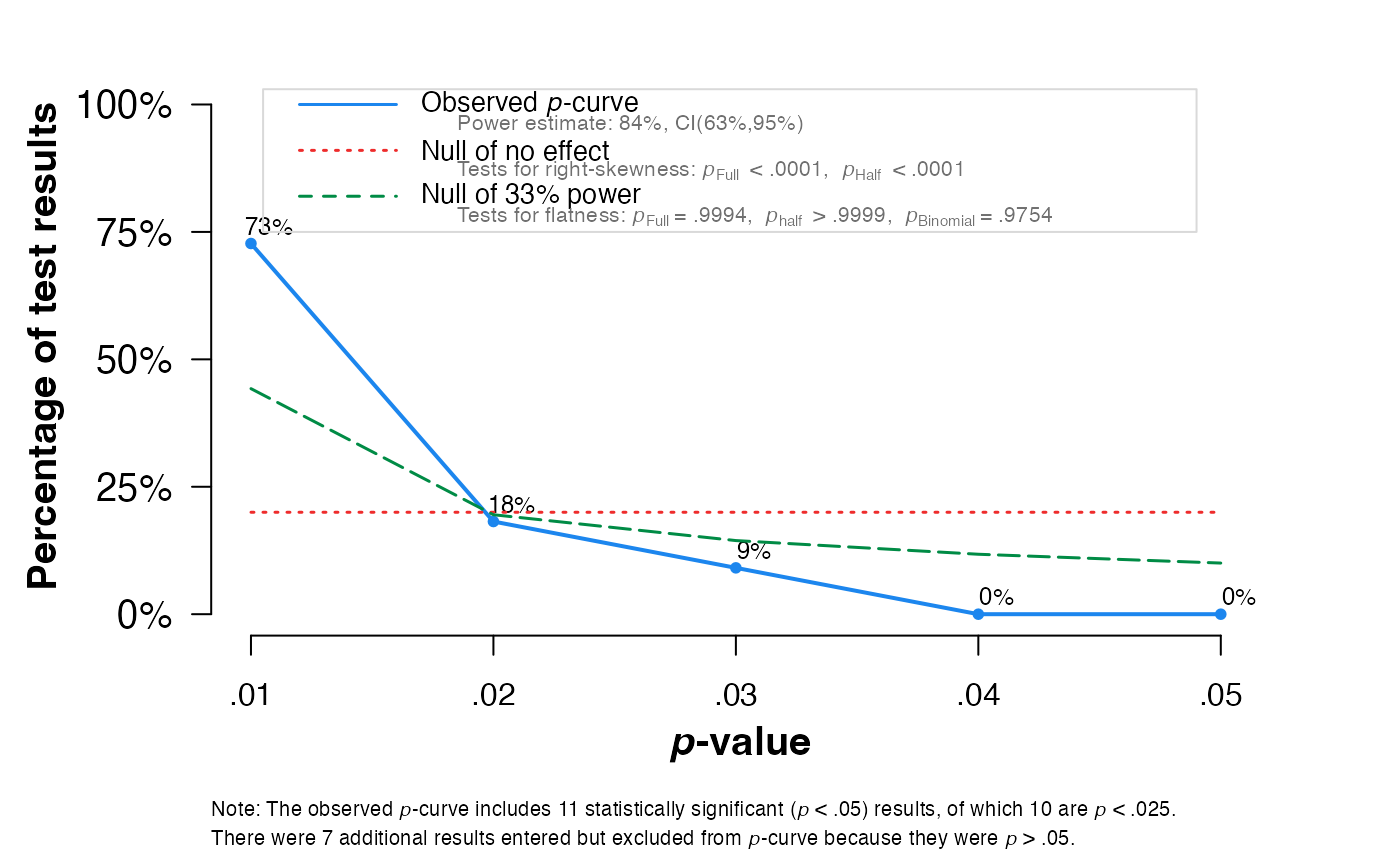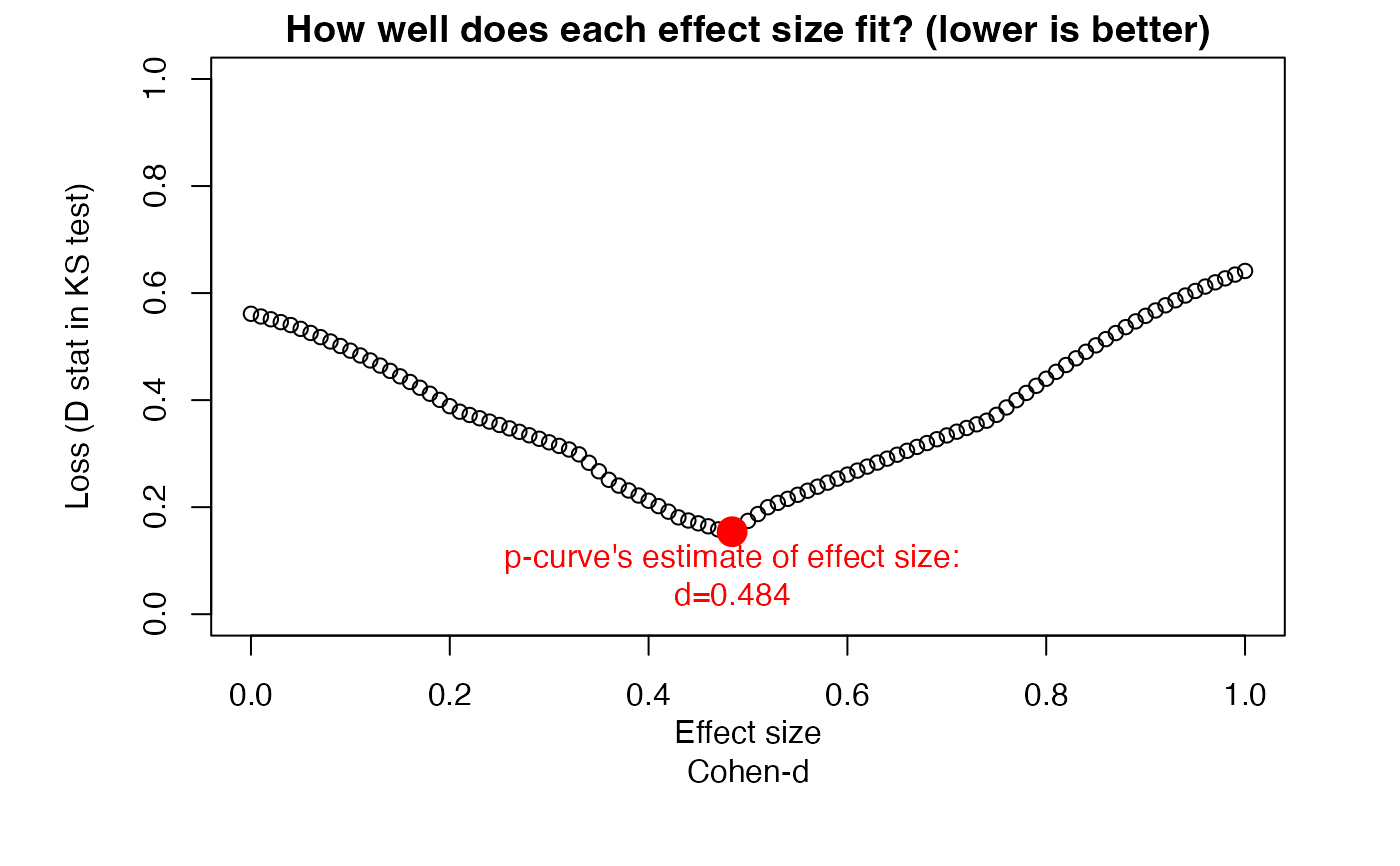#> #> P-curve analysis #> ----------------------- #> - Total number of provided studies: k = 18 #> - Total number of p<0.05 studies included into the analysis: k = 11 (61.11%) #> - Total number of studies with p<0.025: k = 10 (55.56%) #> #> Results #> ----------------------- #> pBinomial zFull pFull zHalf pHalf #> Right-skewness test 0.006 -5.943 0.000 -4.982 0 #> Flatness test 0.975 3.260 0.999 5.158 1 #> Note: p-values of 0 or 1 correspond to p<0.001 and p>0.999, respectively. #> Power Estimate: 84% (62.7%-94.6%) #> #> Evidential value #> ----------------------- #> - Evidential value present: yes #> - Evidential value absent/inadequate: no #> #> P-curve's estimate of the true effect size: d=0.484 #> #> Warning: I-squared of the meta-analysis is >= 50%, so effect size estimates are not trustworthy. # Example 3: Use metacont object, calculate d estimate data("amlodipine") meta2 <- metacont(n.amlo, mean.amlo, sqrt(var.amlo), n.plac, mean.plac, sqrt(var.plac), data=amlodipine, studlab=study, sm="SMD") N = amlodipine$n.amlo + amlodipine\$n.plac
pcurve(meta2, effect.estimation = TRUE, N = N, dmin = 0, dmax = 1)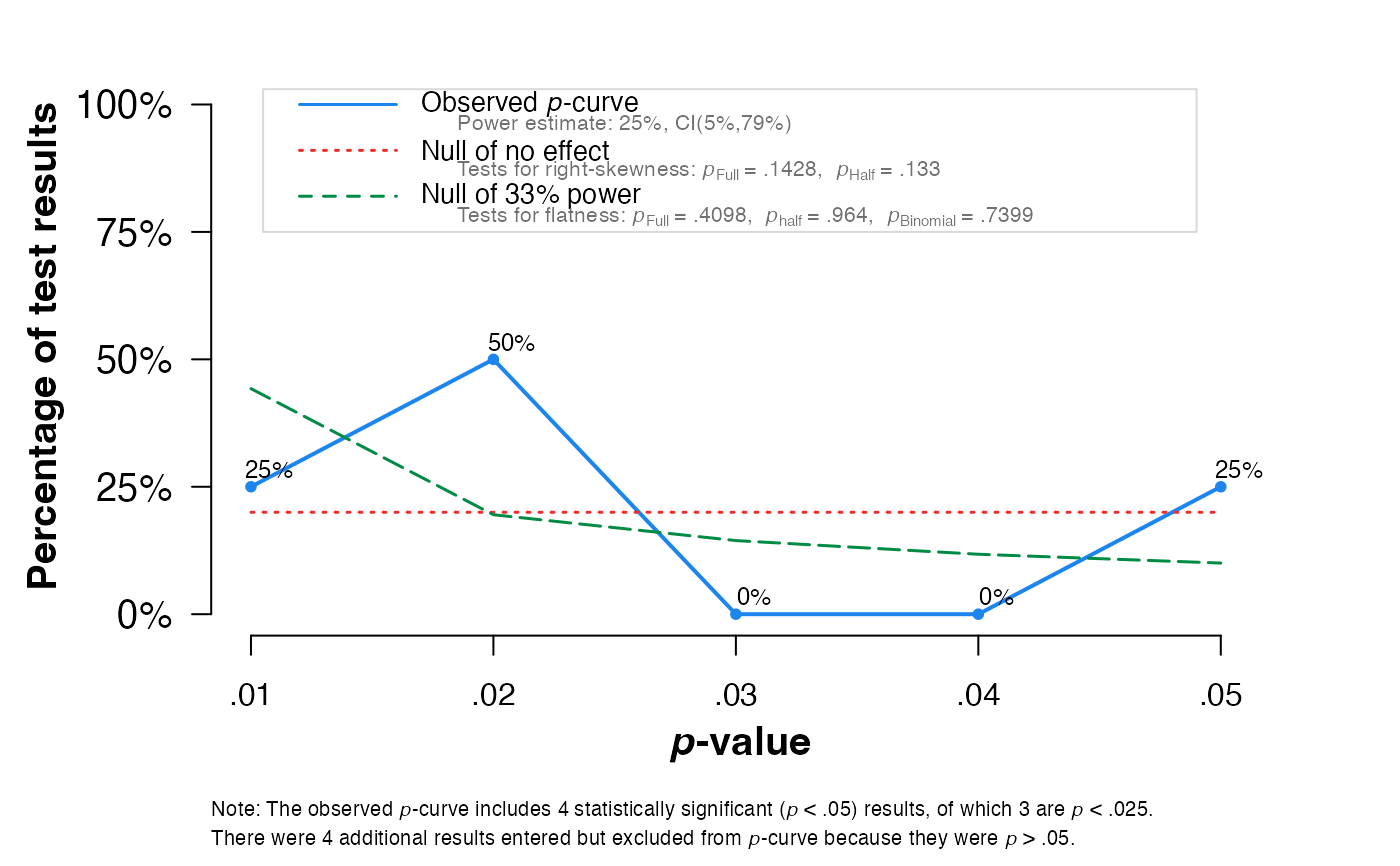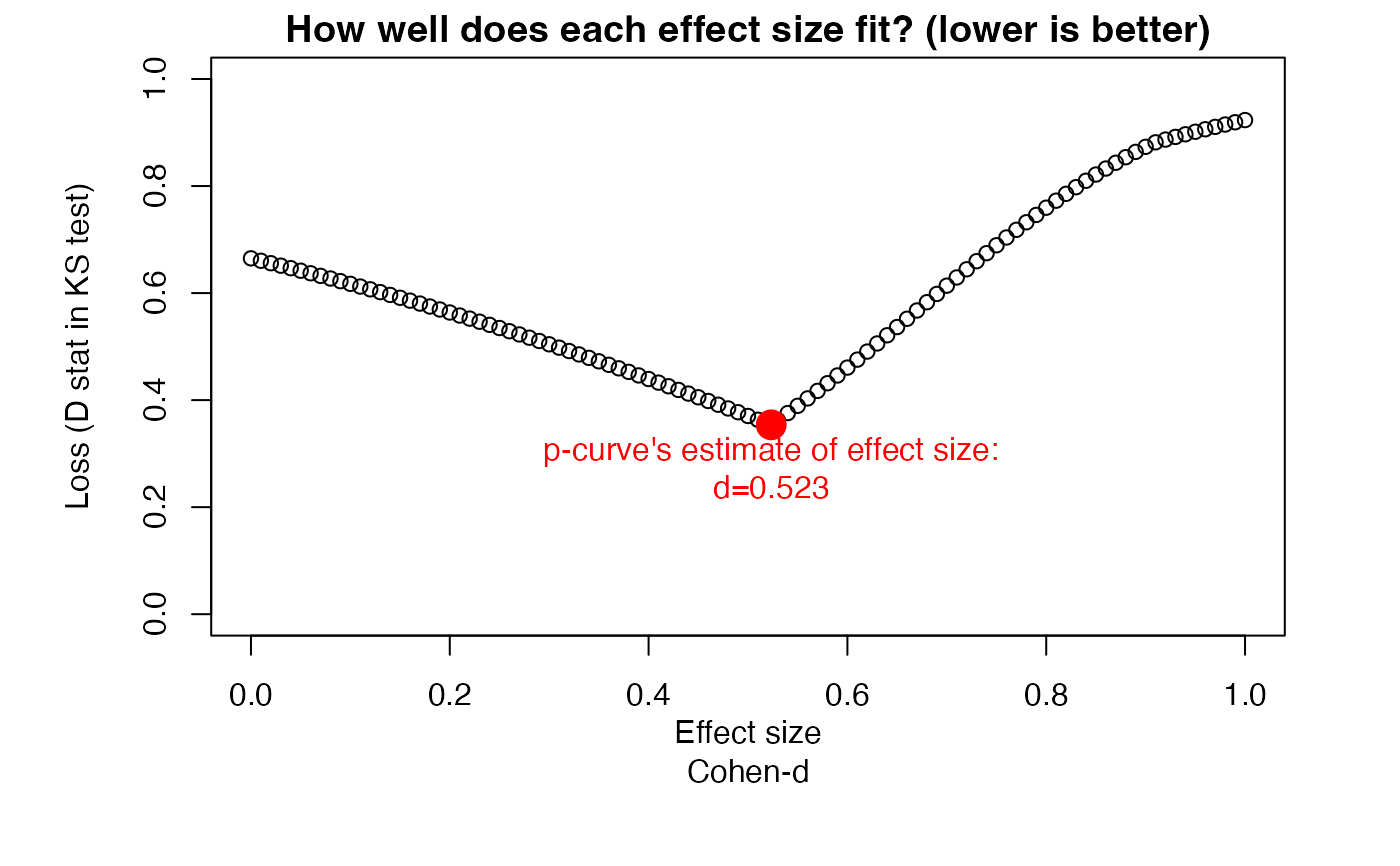#>    #> P-curve analysis
#>  -----------------------
#> - Total number of provided studies: k = 8
#> - Total number of p<0.05 studies included into the analysis: k = 4 (50%)
#> - Total number of studies with p<0.025: k = 3 (37.5%)
#>
#> Results
#>  -----------------------
#>                     pBinomial  zFull pFull  zHalf pHalf
#> Right-skewness test     0.312 -1.067 0.143 -1.111 0.133
#> Flatness test           0.740 -0.229 0.409  1.798 0.964
#> Note: p-values of 0 or 1 correspond to p<0.001 and p>0.999, respectively.
#> Power Estimate: 25% (5%-79.1%)
#>
#> Evidential value
#>  -----------------------
#> - Evidential value present: no
#> - Evidential value absent/inadequate: no
#>
#> P-curve's estimate of the true effect size: d=0.523
# Example 4: Construct x object from scratch
sim = data.frame("studlab" = c(paste("Study_", 1:18, sep = "")),
"TE" = c(0.561, 0.296, 0.648, 0.362, 0.770, 0.214, 0.476,
0.459, 0.343, 0.804, 0.357, 0.476, 0.638, 0.396, 0.497,
0.384, 0.568, 0.415),
"seTE" = c(0.338, 0.297, 0.264, 0.258, 0.279, 0.347, 0.271, 0.319,
0.232, 0.237, 0.385, 0.398, 0.342, 0.351, 0.296, 0.325,
0.322, 0.225))
pcurve(sim)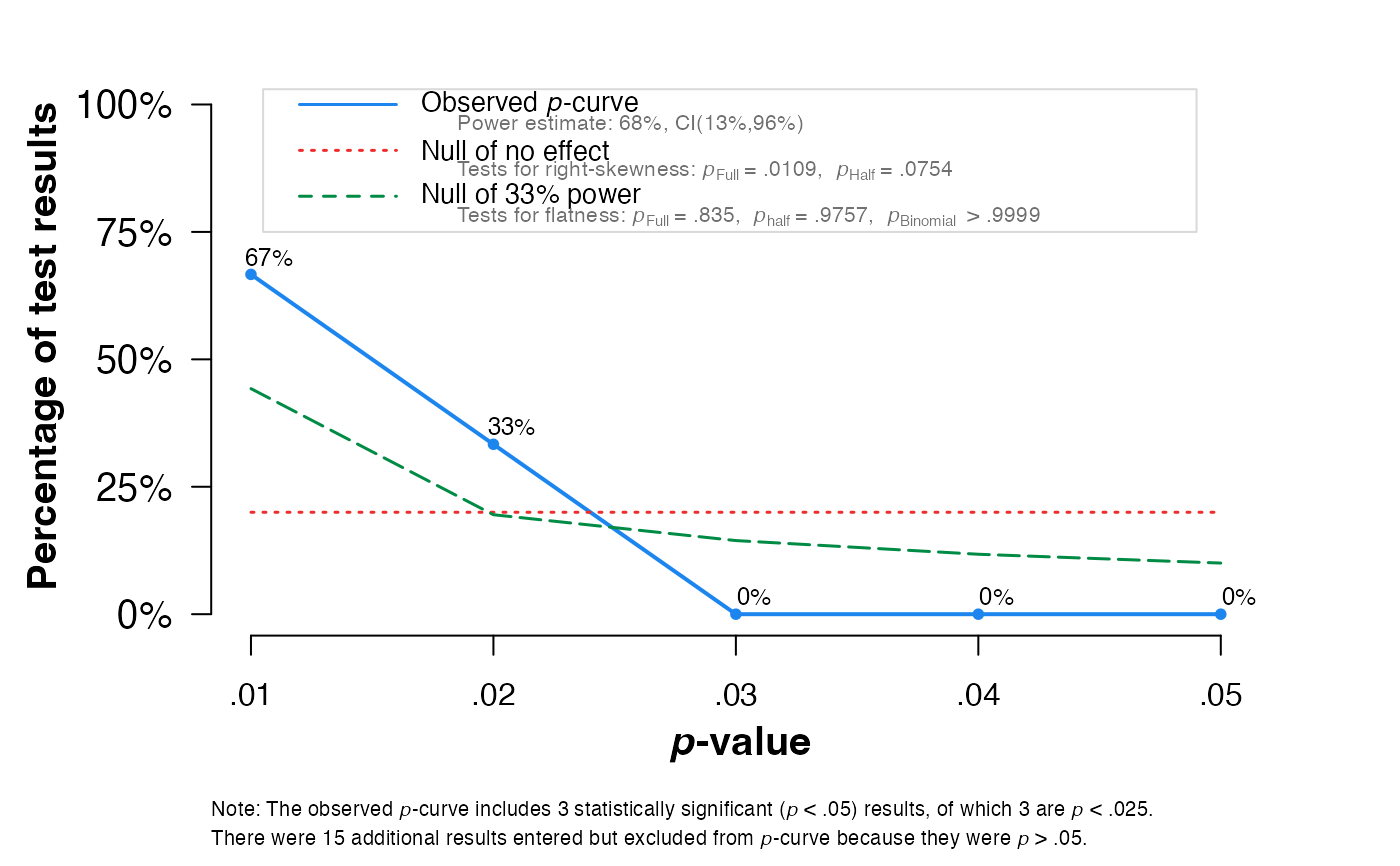#>    #> P-curve analysis
#>  -----------------------
#> - Total number of provided studies: k = 18
#> - Total number of p<0.05 studies included into the analysis: k = 3 (16.67%)
#> - Total number of studies with p<0.025: k = 3 (16.67%)
#>
#> Results
#>  -----------------------
#>                     pBinomial  zFull pFull  zHalf pHalf
#> Right-skewness test     0.125 -2.295 0.011 -1.437 0.075
#> Flatness test           1.000  0.974 0.835  1.971 0.976
#> Note: p-values of 0 or 1 correspond to p<0.001 and p>0.999, respectively.
#> Power Estimate: 68% (13%-95.5%)
#>
#> Evidential value
#>  -----------------------
#> - Evidential value present: yes
#> - Evidential value absent/inadequate: no